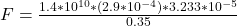## The tibia is a lower leg bone (shin bone) in a human. The maximum strain that the tibia can experience before fracturing corresponds to a 1

Question

The tibia is a lower leg bone (shin bone) in a human. The maximum strain that the tibia can experience before fracturing corresponds to a 1 % change in length.
A. Young’s modulus for bone is about Y = 1.4 x 10 N/m². The tibia (shin bone) of a human is 0.35 m long and has an average cross-sectional area of 2.9 cm. What is the effective spring constant of the tibia?
B. If a man weighs 750 N, how much is the tibia compressed if it supports half his weight?
C. What is the maximum force that can be applied to a tibia with a cross-sectional area, A = 2.90 cm?

in progress 0
6 months 2021-08-08T14:07:51+00:00 1 Answers 5 views 0

## Answers ( )

a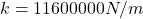b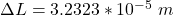c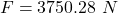Explanation:

From the question we are told that

The Young modulus is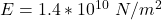The length is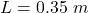The  area is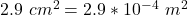Generally the force acting on the tibia is mathematically represented as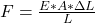derived from young modulus equation

Now this force can also be mathematically represented as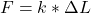So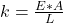substituting values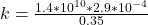Since the tibia support half the weight then the force experienced by the tibia is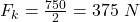From the above equation the extension (compression) is mathematically represented as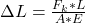substituting values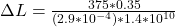From the above equation the maximum force is